# binomial theorem

(redirected from binomial theorems)
Also found in: Dictionary, Thesaurus.

## binomial theorem

a mathematical theorem that gives the expansion of any binomial raised to a positive integral power, n. It contains n + 1 terms: (x + a)n = xn + nxn--1 a + [n(n--1)/2] xn--2a2 +…+ (nk) xn--kak + … + an, where (nk) = n!/(n--k)!k!, the number of combinations of k items selected from n - cf CED3 and CED4 for super/subscript chars
Collins Discovery Encyclopedia, 1st edition © HarperCollins Publishers 2005
The following article is from The Great Soviet Encyclopedia (1979). It might be outdated or ideologically biased.

## Binomial Theorem

(also Newton’s binomial theorem), the name associated with the expansion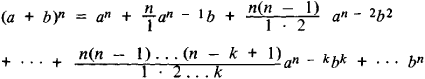where n is a positive integer and a and b are any numbers. In particular,

(a + b)2 = a2 + 2ab + b2

(a + b)3 = a3 + 3a2b + 3ab2 + b3

(a + b)4 = a4 + 4a3b + 6a2b2 + 4ab3 + b4

Similar expressions can be written down for larger values of n.

The coefficients of the binomial formula (1) are called the binomial coefficients; the coefficient of an – kbk is denoted by (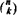) or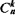. The latter notation is related to combinatorial analysis:is the number of combinations of n different elements taken k at a time.

Binomial coefficients have many remarkable properties: they are all positive integers; the first and last coefficients are equal to unity; the coefficients of terms equidistant from the ends are identical; the coefficients increase from the ends to the middle; and the sum of all the coefficients is equal to 2n. A particularly important property is that the sum of two neighboring coefficients in the expansion of (a + b)n is equal to a certain coefficient in the expansion of (a + b)n+1. For example, the sums 1 + 3, 3 + 3, and 3 + 1 of neighboring coefficients in the formula for (a + b)3 give the coefficients 4, 6, and 4 in the formula for (a + b)4. In general,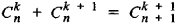Using this property and the known coefficients of (a + b)1 it is possible to obtain the binomial coefficients for any n. The results of the calculations are arranged in a tabular form known as Pascal’s triangle.

The binomial formula for positive integral powers was known long before I. Newton, who in 1676 pointed out the possibility of extending this expansion to the case of a fractional or negative power. A rigorous proof of Newton’s conjecture was given in 1826 by N. H. Abel. In this more general case, the binomial formula begins like (1); the coefficient of an – kbk is given by the expression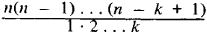In the case of a positive integer n, this expression vanishes for k > n; as a result, formula (1) contains only a finite number of terms. In the case of fractional or negative n, however, all the binomial coefficients are nonzero and the right-hand side of the formula is an infinite series, called the binomial series. If ǀbǀ < a, then this series converges; that is, by taking a sufficiently large number of terms of the series, it is possible to obtain a number as close as desired to (a + b)n. The binomial theorem plays an important role in many branches of mathematics, such as algebra and number theory.